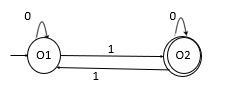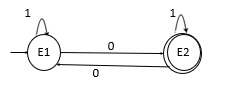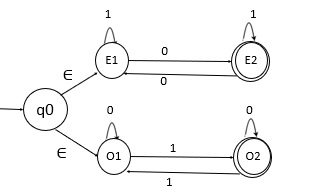# Construct an NFA accepting strings with an even number of 0s or an odd number of 1s

Non-deterministic finite automata (NFA) also have five states which are same as DFA, but with different transition function, as shown follows −

δ: Q X Σ → 2Q

Where,

• Q : Finite set called states.
• Σ : Finite set called alphabets.
• δ : Q × Σ → Q is the transition function.
• q0 ϵ Q is the start or initial state.
• F : Final or accept state.

## Problem

Construct NFA over an alphabet Σ={0,1}.

## Solution

Design two separate machines for the two conditions, as given below −

• NFA accepting only odd number of 1’s

• NFA accepting only even number of 0’s

NFA accepting only odd number of 1’s over an alphabet Σ= {0,1}.

The language it generates is −

L={1,111,01,001,0111,0010,01110,….}

• State o1 on 0 goes to o1 and on 1 goes to state o2.

• State o2 on 0 goes to o2 and on 1 goes to o1.NFA Accepting only even number of 0’s over an alphabet Σ= {0,1}

The language it generates is −

L={100,1100,1010,1100010,…….}

• State E1 on 1 goes to E1 and on 0 goes to E2.

• State E2 on 1 goes to E2 only and on 0 goes to E1.Now merge the two transition diagrams with an epsilon move.

The NFA that accepts all strings with an even number of 0s or an odd number of 1s is as follows −